# Formatting Time - Hours: Minutes

Wikitechy | 1341 Views | javascript | 04 Jun 2016

• In JavaScript the Hours and minutes are obtained by using getHours() and getMinutes() methods of the date object.
• The hour’s values will be varied from 0 to 23.
• In JavaScript there are two problems that occur while returning the data value
• The time in hour or minutes are return in single digits which indicates that the JavaScript does not add any leading zeroes which is nothing but the prefix value. Hence, 05:03 (AM) is printed as 5:3.
• The time 12:00 AM is printed as 0:00.

#### Sample Code :

```<script type="text/javascript">
var currentdate = new Date();
var curr_hour = currentdate.getHours();
var curr_min = currentdate.getMinutes();
document.write(curr_hour + " : " + curr_min);
</script>```

####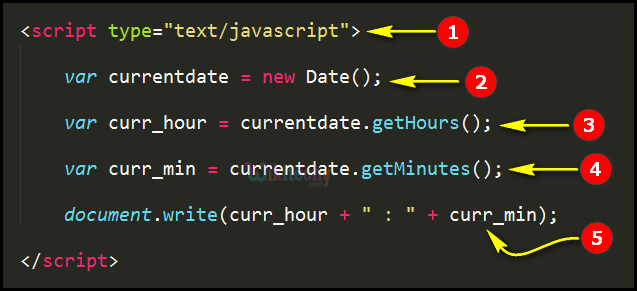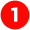Generally, the <script> tag is mainly used for defining the client-side script (JavaScript). In this statement the script element "type" attribute defines the media type as "text".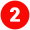Here in this statement we create an object(currentdate) for the date method using new operator, where the current date will be stored in the variable “currentdate”.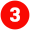In this statement we get the current hour by using the getHours function with reference of object “currentdate” where the current hour’s value will be stored in the variable “curr_hour”.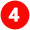Similarly, in this statement we get the current minute by using the getMinutes function with the reference of object “currentdate” where the current minute’s value will be stored in the variable “curr_min”.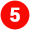Here in this statement “document. Write” function is used to write the current hours & minutes in the browser window by using the concatenation operator” +”.

####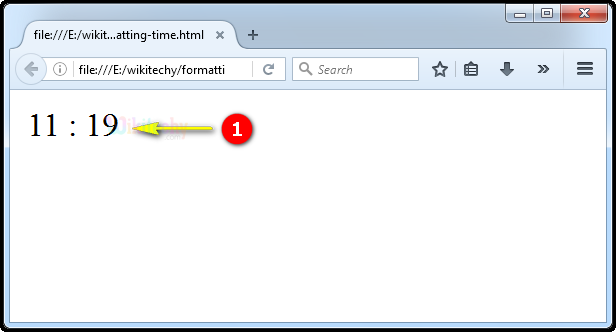Here in this output display the current time as “11:19” that is eleven hours and nineteen minutes as shown.

Bug Bounty
Webinar